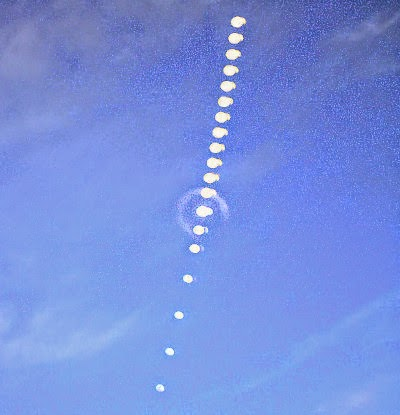### Star trails

Star trail pictures are created by merging multiple images together into one image.Moon trail
The principle behind creating star trail pictures is very simple, but can also be very complex. We are not getting into the complex one in this post, which makes guesses and predictions to feel the gaps between stars, to keep the background static, etc...

In this post we're taking a look at the simplest way of creating star trails, which is by keeping the most intensive pixel colors from a list of images.

Each pixel has a RGB value, which can range from black (0,0,0) to white (255,255,255). The first column is for red, the second column is for green and the last one is for blue, and hence the RGB acronym.

The intensity of a pixel color can be calculated with the following formula: (R+G+B)/3.

But there are some other formulas for calculating the brightness of a pixel, which also work for creating star trails:
• HSL Lightness
• `0.5*max(R,G,B) + 0.5*min(R,G,B)`
• Relative luminance
• `0.2126*R + 0.7152*G + 0.0722*B`
• Luma
• `0.299*R + 0.587*G + 0.114*B`
• `sqrt(0.299*R^2 + 0.587*G^2 + 0.114*B^2)`

On this principle, a new Perl script has been created which reads a list of images and stores the pixel colors inside a matrix, which is updated when a more intensive pixel color is found.

In the end, it creates a new image and sets the pixels to the colors stored inside the matrix.

Implementations:
Bonus: